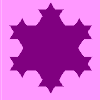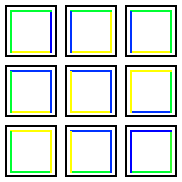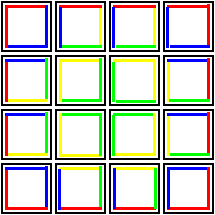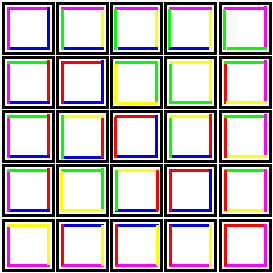#### You may also like### Is There a Theorem?

Draw a square. A second square of the same size slides around the first always maintaining contact and keeping the same orientation. How far does the dot travel?### Coins on a Plate

Points A, B and C are the centres of three circles, each one of which touches the other two. Prove that the perimeter of the triangle ABC is equal to the diameter of the largest circle.### Smaller and Smaller

Can you predict, without drawing, what the perimeter of the next shape in this pattern will be if we continue drawing them in the same way?

# On the Edge

##### Age 11 to 14Challenge Level

Adam of Moorside School sent us in his description of a possible painting for the three by three square:

The outside of the square should be green. The rest of one of the corner squares should be yellow. The rest of another corner square should be blue. The other two corner squares should have one side blue and the other side yellow. Then two of the squares in between the corner squares should have two touching sides yellow and one side blue. The other two squares in between the corner squares should have two touching sides blue and one side yellow. The middle square should be half yellow, half blue.I noticed that in an $n \times n$ square there are a total on $n^2$ small squares, and therefore a total of $4 n^2$ edges to be coloured. Each colour takes up $4 n$ of these edges, so it should be possible to colour an $n \times n$ square with $n$ different colours. To do this, we need to arrange for all the colours to have four cornerpieces and $4 (n-2)$ edge pieces."